## Introduction

Bootstrap percolation was originally introduced by Chalupa, Leath and Reich1 in the context of magnetic disordered systems in 1979. Since then, it has been studied extensively by physicists and sociologists, mainly due to its connections with various physical models and a variety of applications such as neuronal activity2 and jamming transitions3. Bootstrap percolation can be essentially considered as an activation process on networks: (i) Nodes are either active or inactive; (ii) Once activated, a node remains active forever; (iii) Initially, each node is in an active state with a given probability p; (iv) Subsequently, inactive nodes become active if they have at least k active neighbors; (v) Nodes are activated in an iterative manner according to the condition in (iv), until no more nodes can be activated. This process has been investigated on different kinds of networks including lattices4,5, trees6,7, random networks8,9,10,11 and so on.

Bootstrap percolation has found applications in explaining many important social phenomena, such as the spreading of information12, the propagation of infection13,14, the adoption of new products and social behaviors15,16,17,18 including trends, fads, political opinions, belief, rumors, innovations and financial decisions. For instance, in emergence of cultural fads and adoption of new technology or objects, an individual can be positively influenced when there is a sufficient number of its close friends who have also done so19. In other words, one may decide to buy a product when recommended by at least k users and trust a message when told by at least k neighbors; cf. the well-known rule, “What I tell you three times is true”20. In this way, the process leads initially localized effects propagating throughout the whole network. Moreover, a broad range of generalized formulations of bootstrap percolation on social networks are investigated, such as Watts’ model of opinions21, in which k is replaced by a certain fraction of the neighbors and disease transmission models with different degrees of severity of infection22.

Real networks are often embedded in space23 and social networks are no exception. Previous empirical studies of online social networks24, email networks25 and mobile phone communication networks26 have confirmed a spatial scaling law, namely, the probability density function (PDF) of an individual to have a friend at distance r scales as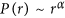,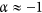27. In fact, prior to these empirical observations, Kleinberg28 has proposed a spatial network model by adding long-range links to a 2-dimensional lattice and he has proved that when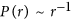, the structure is optimal for navigation. Recently, Hu et al.27 suggested the optimization of information collection as a possible explanation for the origin of this spatial scaling law.

Extensive studies have shown that the spatial organization can change the dimension29,30,31,32,33, which dominates many important physical properties of networks34,35,36,37,38,39,40,41. Moukarzel et al.42 studied k-core percolation on long-range spatial networks, which is built by taking a 2-dimensional lattice and adding to each node one or more long-range links. In the networks, the probability density function (PDF) of long-range links with length r scales as. By numerical simulation, they found that the 3-core transition is of first-order for α > −1.75 (it is equivalent to the scaling of 2.75 found by Moukarzel et al.42) and of second-order for smaller α. In fact, k-core percolation has close relation to bootstrap percolation43,44, nevertheless the two processes have different features from each other, being strongly dependent on the network structure8,45. Although there is a deeper understanding of percolation processes and spatial networks now, how spatial organization influences the spreading process on social networks under the framework of bootstrap percolation remains further investigation.

In this paper, we numerically study bootstrap percolation on undirected Kleinberg’s spatial networks, which is a typical artificial social network. Setting the relative size of the giant active component to the network size as the order parameter, we find that the distribution of long-range links’ lengths can change the order of phase transition. In particular, our main findings are as follows: (i) We find a parameter-dependent critical value αc, above which a double phase transition46 is observed. Here, the so-called double phase transition means a mixture of two transitions at different critical points, consisting of a hybrid phase transition and a second-order one. In this paper, we use the hybrid phase transition to indicate a first-order phase transition in which the order parameter has a discontinuous jump between two non-zero values. (ii) Surprisingly, we find a parameter-independent critical value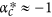, about which the two critical points for the double phase transition are almost constant. (iii) When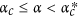, the first-order critical point decreases and the second-order critical point increases as α decreases. When α < αc, there is only a second-order phase transition with an increasing critical point as the decreasing of α. Furthermore, we test the universality of our findings by drawing the phase diagram and give a possible explanation of the rich phase transition phenomena by simulating on related networks. Our findings indicate that the spatial scaling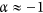, observed in real social networks, may be resulted from some deep-going principles in addition to the optimization of navigation and information collection, which is not yet fully understood now.

## Results

Kleinberg model28 is a typical spatial network model, which has been well justified by empirical data24,25,26. Here, the undirected Kleinberg’s spatial network is constrained on a 2-dimensional periodic square lattice consisting of N = L × L nodes. In addition to its initially connected four nearest neighbors, each node i has a random long-range link to a node j with probability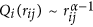, where α is a tunable exponent and rij denotes the Manhattan distance, which quantifies the length of the shortest path between node i and node j, following strictly the horizontal and/or vertical links in lattices. Since the number of nodes at distance r to a given node is proportional to rd−1 in a d-dimensional lattice, the probability Q(rij) can be mapped to a probability density function (PDF),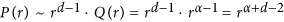. In the present 2-dimensional case, where d = 2, the probability density function(PDF) scales as. An illustration of a 2-dimensional undirected Kleinberg’s spatial network can be found in Fig. 1.

In the following, we focus on three indicators: (i) The relative size of the giant active component (Sgc) at the equilibrium, i.e., the probability that an randomly selected node belongs to the giant active component; (ii) The number of iterations (NOI) to reach the equilibrium, which is usually used to determine the critical point for the first-order phase transition47,48; (iii) The relative size of the second giant active component (Sgc2), which is usually used to determine the critical point for the second-order phase transition32,48.

Figure 2 shows rich phase transition phenomena when taking Sgc as the order parameter. When α ≥ −1, the curves of Sgc(p) are well overlapped and the system undergoes a double phase transition, mixed of a hybrid phase transition and a second-order one as shown in Fig. 2a. Notice that Sgc has a continuous increase at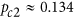(the second-order critical point), where the transition is of second-order. In contrast, Sgc has an abrupt jump directly from around 0.58 to almost 1 at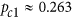(the first-order critical point), where there is a hybrid phase transition. Surprisingly, the two critical points seem to be constant when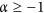, as indicated by the four overlapped Sgc(p) curves in Fig. 2a. When α < −1, there is only a second-order phase transition with an increasing pc2 as the decreasing of α (see Fig. 2b). Specifically,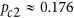when α = −2 and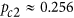when α = −5. Although Sgc goes up sharper after p exceeds pc2 as α getting smaller, simulations justify that the curve of Sgc(p) is still continuous, meaning that the transition is indeed of second-order when α < −1.

Finding critical points via simulations is always a difficult task that requires high precision. When α ≥ −1, where a part of the double phase transition is a hybrid phase transition, we can determine the critical point pc1 by calculating the number of iterations (NOI) in the cascading process, since NOI sharply increases when p approaches pc1 for the first-order phase transitions47,48. Accordingly, pc1 is calculated by plotting NOI as a function of p. As shown in Fig. 2c, NOI reaches its maximum at the same p when α ≥ −1, which is the evidence thatis almost a constant value. Analogously, by plotting Sgc2 as a function of p, we can precisely identify pc232,48, at which Sgc2 reaches its maximum (see Fig. 2d). We can see that pc2 increases as α decreases, as(α ≥ −1), 0.176 (α = −2) and 0.256 (α = −5).

Although to justify the hybrid phase transition and to determine the critical value αc by simulations in a finite discrete system are not easy, we solve this problem by a cross-validation on the critical point pc1 and the critical value αc. Firstly, we fix α = −1 to determine pc1. On the one hand, there is an intersection for curves of Sgc(p) at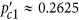under different network sizes as shown in Fig. 3a, which can be considered as the critical point according to the finite-size analysis48. On the other hand, the corresponding NOI reaches its maximum atwhen L = 800 as shown in Fig. 3b. Combining these two observations, a more appropriate critical point is identified as the average value. Conversely, we fix p = 0.263 to determine αc. From Fig. 3c, we can see that Sgc has two phases: about 0.58 or close to 1 when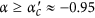, which is a strong evidence that Sgc undergoes a hybrid phase transition. If the increasing of Sgc is continuous, there is no such gap between the two phases. From Fig. 3d, we note that the corresponding averaging NOI reaches its maximum at. Combining these two evidences, we appropriately identify the critical value as the average value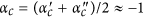.

In addition, pc1 for the hybrid phase transition should be almost constant when α ≥ αc, otherwise we cannot observe the separation of two phases in Fig. 3c for a fixed value p = 0.263. To verify that, we estimate errors of pc1 by varying α under fixed network size L. As shown in Fig. 4a, pc1 slightly decreases when α approaches the critical value. Even though, when α is in the range [−1,4], the difference between maximum and minimum value of pc1 is only 0.0008 after over 1000 realizations, which is very small compared to the whole range of p (i.e. [0,1]), indicating that the value of pc1 is not sensitive to the parameter α when α ≥ −1. Based on these evidences, pc1 can be roughly considered as a constant and its value is about 0.2634, which is the mean value of pc1 when α in the range [−1,4]. Furthermore, taking α = −1 as an example, we consider the effects of finite-size of networks on these results. As shown in Fig. 4b, the mean value of pc1 gradually approaches an extreme value around 0.263 and the standard deviation of pc1 decreases as L goes to infinity. Similar results hold for the analysis of the critical point pc2 and its value is also almost constant as 0.134.

A representative phase diagram for Sgc in the p − α plane is shown in Fig. 5. We find that the varying of α, which dominates the distribution of long-range links’ lengths, can change the order of phase transition. Overall,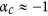is confirmed to be a critical value, above which a double phase transition (region II) is present. When α ≥ −1, the curves of Sgc(p) are overlapped, suggesting that the properties of bootstrap percolation on these spatial networks are alike. When α < −1, the hybrid phase transition vanishes and Sgc only undergoes a second-order phase transition (region I) with an increasing critical point as the decreasing of α. The maximum of pc2 is about 0.259, which is obtained when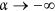, i.e., all long-range links’ lengths are 2.

To test the universality of the findings, we simulated on undirected Kleinberg’s spatial networks in parameter spaces (k, α, kl) and determined the critical points. Results are shown in Fig. 6. According to the relationship between the threshold k and half of the average degree of the network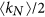, where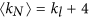, there are three regions in the phase diagram:

• When k is remarkably smaller than, e.g, k = 1 compared to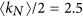, there is only a trivial first-order phase transition at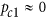.

• When k is around, e.g., k = 3 compared to, there is a critical value αc, above which a double phase transition is observed. The value of αc depends on the choice of both k and kl. In particular,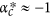is found to be a parameter-independent critical value, about which the two critical points for the double phase transition are almost constant. Specifically, as shown in the phase diagram of Fig. 6, the color of data points for the same parameter k is nearly unchanged when α ≥ −1, which is a strong evidence that the values of pc1 and pc2 are almost constant. When, pc1 decreases and pc2 increases as α decreases. Note that αc can be equal toin some parameter spaces, such as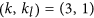and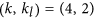.

• When k is remarkably larger than, e.g, k = 5 compared to, the hybrid phase transition is absent and Sgc only undergoes a second-order phase transition with an increasing pc2 as the decreasing of α (see Supplementary Figs S1-S3 for the detailed shapes of Sgc(p) curves).

Moreover, simulations confirm that our main results also hold for Kleinberg’s spatial networks with directed long-range links since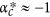is still a critical value. However, there is only a first-order phase transition with pc1 being almost constant instead of the formal double transition when α ≥ −1 (see Supplementary Fig. S4). In addition, simulations on undirected Kleinberg’s spatial networks without periodic boundary conditions suggest that whether the square lattice has periodic boundary conditions does not essentially affect our main results (see Supplementary Fig. S5).

To provide the insights on the mechanism of the transition, we simulate on different networks and compare with other related transitions. These networks include a simple 2-dimensional lattice (Lattice), networks with all 5 links being long-range (LR) and networks without spatial structure, i.e. random 5-regular networks (RR). In the LR network, which is a special case of long-range percolation model in the 2-dimensional space49,50,51, each node is associated with only kl = 5 undirected long-range links instead of initially connected short-range links based on a 2-dimensional periodic lattice. As shown in Fig. 7, the curves of Sgc(p) on the spatial networks are between the ones on Lattice network and RR network. When α = −4, the Sgc(p) curve on the spatial network has similar trend with the one on Lattice network since the very long-range links are rare and the transition is of second-order. When α ≥ −1, there is a double phase transition and the curves of Sgc(p) are almost overlapped with the one on RR network. These observations indicate that, to turn the value of α, we can change the bootstrap percolation properties of spatial networks from Lattice network to RR network, or vice versa. More specifically, when α = −4, all long-range links are highly localized and the structure of spatial networks is similar to Lattice network, whereas when α ≥ −1, mainly due to the existence of very long-range links, the spatial networks behave like RR network.

Together, it should be noted that the Sgc(p) curve on LR network when α = −1 acts like the ones on RR network and spatial networks when α ≥ −1. To better understand how does α affect the transition on LR network, taking kl = 5 as an example, we show the phase diagram after k = 3 bootstrap percolation in Fig. 8. The diagram is divided into three regions by critical values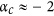and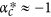. As α decreases, the transition is of second-order with an increasing pc2 when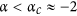(region I). There is a double phase transition when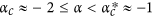(region II), where pc1 decreases and pc2 increases as α decreases. Once again, a double phase transition with two almost constant critical points,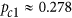and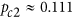, is observed when(region III). Further simulations suggest that similar main results also hold under other combinations of k and kl (see Supplementary Fig. S6 for the phase diagram and Supplementary Figs S7–S11 for the shapes of Sgc(p) curves).

In fact, for the original Kleinberg’s spatial networks with directed long-range links, Sen et al.52 found that the varying of α can change the network structure, namely, the network is regular-lattice-like when α < −2, small-world-like when −2 < α < −1 and random-like when α >−1. More recent studies30,31,32 also proposed three regimes: (i) When α > −1, the dimension of the spatial network is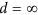and the percolation transition belongs to the university class of percolation in Erdös-Rényi networks. (ii) When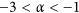, d decreases continuously fromto d = 2 and the percolation shows new intermediate behavior. (iii) When α < −3, the dimension is d = 2 and the percolation transition belongs to the university class of percolation in regular lattices. These previous findings suggest that the properties of spatial networks have qualitative changes when α is around −1, which is corresponding to the observation of these phase transition phenomena here.

## Discussion

In summary, we have studied bootstrap percolation on spatial networks, where the distribution patterns of long-range links’ lengths can change the order of phase transition. In particular, we find a parameter-dependent critical value αc, above which a double phase transition, mixed of a hybrid phase transition at a higher p and a second-order phase transition at a lower p, is present. It is particularly interesting that we find a almost parameter-independent critical value, about which the curves of Sgc(p) are well overlapped, indicating that the two critical points for the double phase transition are almost constant when α ≥ −1. As bootstrap percolation has not been studied yet on undirected Kleinberg’s spatial networks, our novel findings indicate that the topological properties of undirected Kleinberg’s spatial networks are alike when α ≥ −1 in the 2-dimensional space. In fact, the scaling lawhas been empirically observed in many real networks24,25,26, which may be resulted from complex self-organizing processes toward optimal structures for information collection27 and/or navigation28. Since the cascading processes on spatial networks are almost the same when α ≥ −1,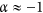is indeed corresponding to the structure with the smallest average geographical length of links, which can exhibit as effective spreading of information as networks with even longer shortcut links. This is to some extent relevant to the principle of least effort in human behavior53.

We find the varying of α can change the bootstrap percolation critical behavior from random regular networks to lattices, or vice versa. In particular, when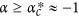, the spatial networks behave like random regular networks and there is a double phase transition. When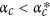, there is a richer phase transition phenomena. More specifically, the double phase transition is still present when, however, instead of being constant, the first-order critical point decreases and the second-order critical point increases as α decreases. The observation of such results may be mainly due to the small-world-like network structure52,54, which leads the transition showing a intermediate behavior. When α < αc, the hybrid phase transition vanishes and there is only a second-order phase transition with an increasing critical point as the decreasing of α. When α goes to negative infinity, where all long-range links are highly localized, the spatial networks degenerates into regular lattices and the transition is of second-order. In this way, we give a possible explanation of the emergence of these phase transition phenomena.

Moreover, our results are, to some extent, relevant to the control of information spreading. For example, when α ≥ αc, if we would like to make as many people as possible to know the information, the optimal choice of the fraction of initially informed people should be pc1, since larger initially informed population provides no more benefit but requires higher cost as shown in Fig. 2a. Therefore, the results help us in better understanding the self-organization of spatial structure of online social networks, in terms of the effective function for information spreading. Besides, our work can possibly find applications in studying the diffusion of virus spread in other spatial networks such as ad hoc, wireless sensor networks and epidemiological graphs. However, in the context of a numerical study, it is really hard to tell whether the critical value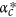is exactly −1 and whether the critical points are completely independent of α when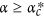. In addition, how does the transition depend on the spreading processes and what kind of transitions does it belong to for real social networks are still open questions. Hence, we expect to verify our findings in an analytical way and based on other generalized network models, including spatially constrained Erdös-Rényi networks55, network of networks (NON)56, multiplex networks57 and real social networks. Besides, to assign each node one long-range link is a high-cost strategy when generating artificial spatial social networks, we leave individualized number of long-range links associated with each node and partial spatial embedding as future works.

## Methods

To numerically implement the spatial scaling α when generating Kleinberg’s spatial networks, we add undirected long-range links to a 2-dimensional periodic square lattice in a smart way as follows. First, a random length r between 2 and L/2 is generated with probability, which ensures the scaling in advance. Second, random segmentations of length r to Δx and Δy with the only constraint that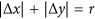are done to determine candidate nodes, where Δx and Δy are both integers. Namely, for an uncoupled node i with coordinates (x, y), named target node, all candidate nodes are these with coordinates (x + Δx, y + Δy) such that. The above procedure ensures all candidate nodes at distance r from the target node i are uniformly distributed. Hence, we can randomly choose an uncoupled candidate node (i.e., a node without any long-range link) to link with target node i. Be noted that, on a large system, a finite fraction of the nodes will have all candidate nodes already connected when α is very small. To deal with this problem, we additionally adopt an alternate procedure referring to the distance coarse graining procedure58, in which we randomly choose an uncoupled nearest neighbor node of these candidate nodes until the linking is accomplished. We repeat such procedure for the rest uncoupled nodes until each node of the network has one undirected long-range link such that the degree of each node is exactly 5.